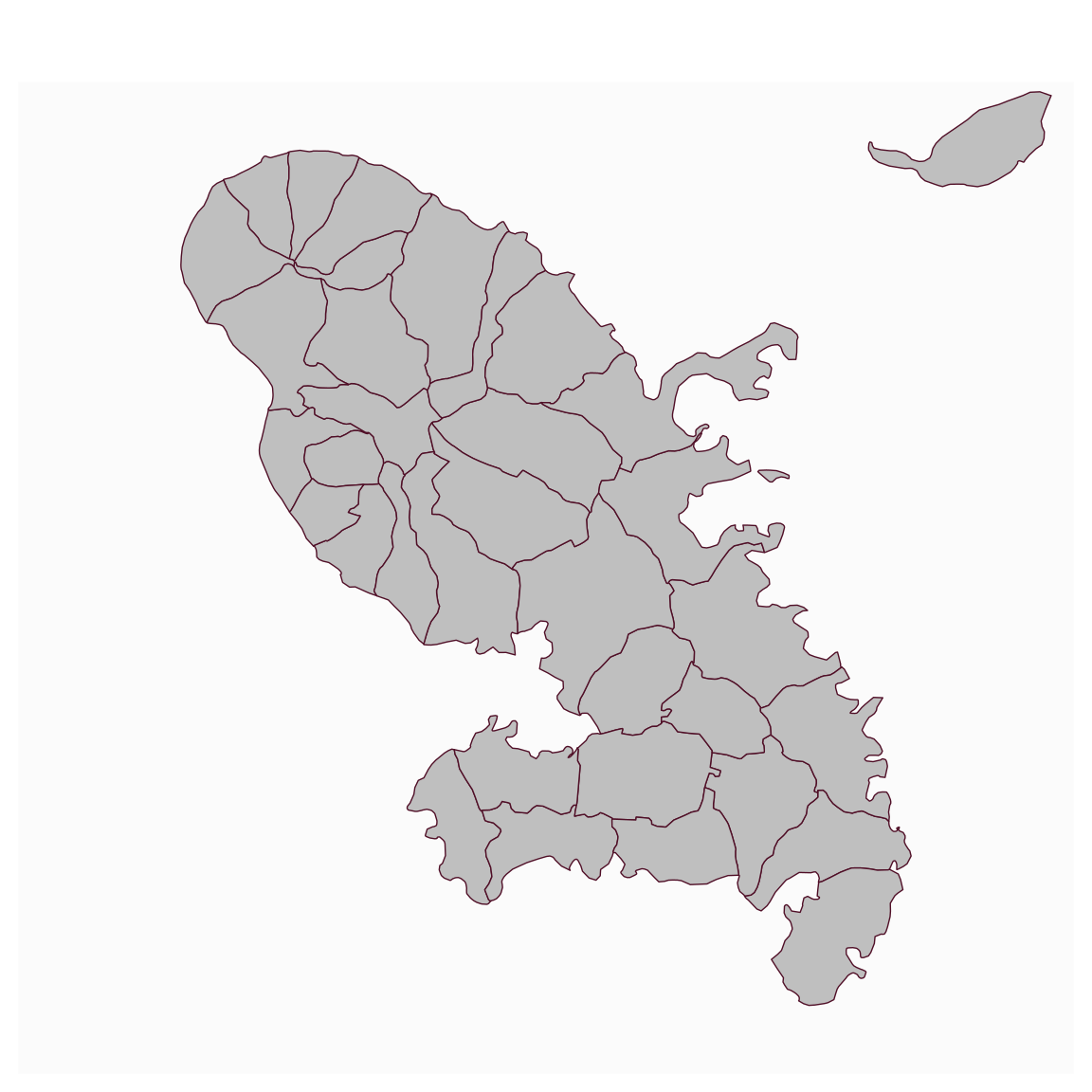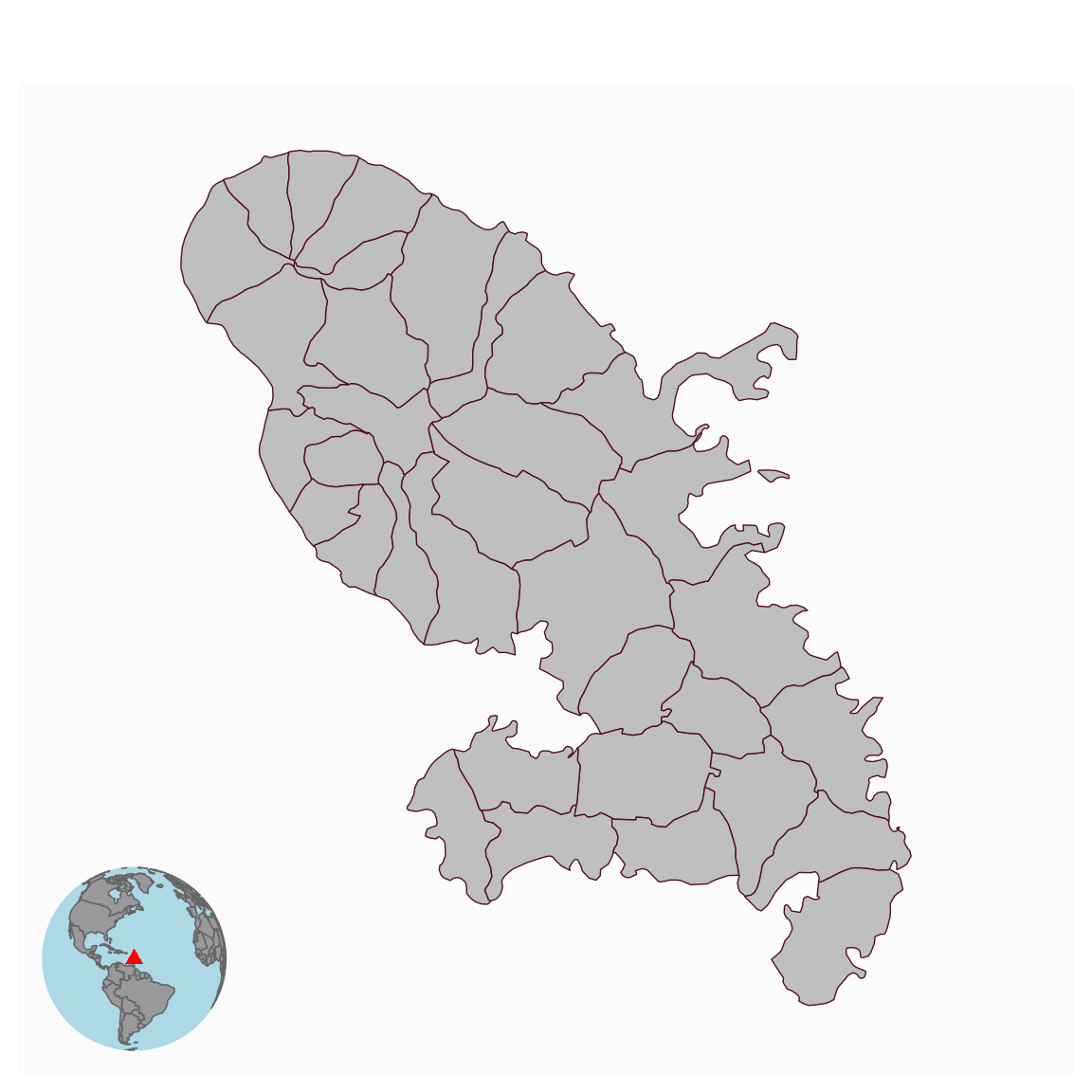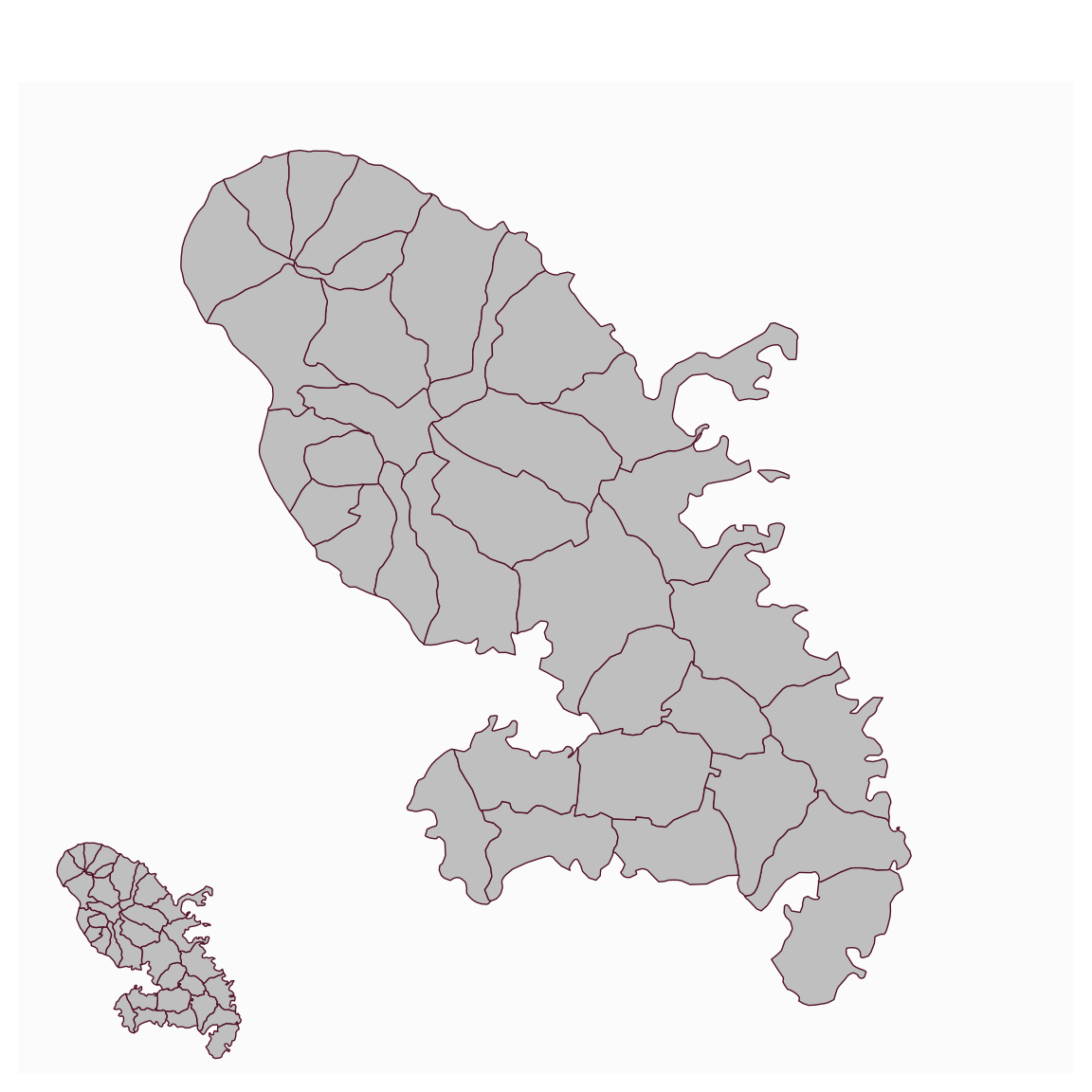This function is used to add an inset map to the current map.

mf_inset_on(x, pos = "topright", cex = 0.2, fig)

mf_inset_off()

## Arguments

x

an sf object, or "worldmap" to use with mf_worldmap.

pos

position, one of "bottomleft", "left", "topleft", "top", "bottom", "bottomright", "right", "topright"

cex

share of the map width occupied by the inset

fig

coordinates of the inset region (in NDC, see in ?par())

## Value

No return value, an inset is initiated or closed.

## Details

If x is used (with pos and cex), the width/height ratio of the inset will match the width/height ratio of x bounding box.
If fig is used, coordinates (xmin, xmax, ymin, ymax) are expressed as fractions of the mapping space (i.e. excluding margins).
If map layers have to be plotted after the inset (i.e after mf_inset_off()), please use add = TRUE.
It is not possible to plot an inset within an inset.
It is possible to plot anything (base plots) within the inset, not only map layers.

## Note

This function does not work when mfrow is used in par().

## Examples

mtq <- mf_get_mtq()
mf_map(mtq)
mf_inset_on(x = mtq[1, ], cex = .2)
mf_map(mtq[1, ])
mf_inset_off()mf_map(mtq)
mf_inset_on(x = "worldmap", pos = "bottomleft")
mf_worldmap(x = mtq)
mf_inset_off()mf_map(mtq)
mf_inset_on(fig = c(0, 0.25, 0, 0.25))
mf_map(x = mtq)
mf_inset_off()